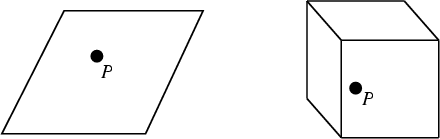## PointA 0-Dimensional mathematical object which can be specified in-D space usingcoordinates. Although the notion of a point is intuitively rather clear, the mathematical machinery used to deal with points and point-like objects can be surprisingly slippery. This difficulty was encountered by none other than Euclidhimself who, in his Elements, gave the vague definition of a point as that which has no part.''

The basic geometric structures of higher Dimensional geometry--the Line, Plane, Space, and Hyperspace--are all built up of infinite numbers of points arranged in particular ways.

See also Accumulation Point, Antigonal Points, Antihomologous Points, Apollonius Point, Boundary Point, Branch Point, Brianchon Point, Brocard Midpoint, Brocard Points, Cantor-Dedekind Axiom, Center, Circle Lattice Points, Concur, Concurrent, Congruent Incircles Point, Congruent Isoscelizers Point, Conjugate Points, Critical Point, Crucial Point, Cube Point Picking, Cusp Point, de Longchamps Point, Double Point, Eckardt Point, Elkies Point, Elliptic Fixed Point (Differential Equations), Elliptic Fixed Point (Map), Elliptic Point, Equal Detour Point, Equal Parallelians Point, Equichordal Point, Equilibrium Point, Equiproduct Point, Equireciprocal Point, Evans Point, Exeter Point, Exmedian Point, Fagnano's Point, Far-Out Point, Fejes Tóth's Problem, Fermat Point, Feuerbach Point, Feynman Point, Fixed Point, Fletcher Point, Gergonne Point, Grebe Point, Griffiths Points, Harmonic Conjugate Points, Hermit Point, Hofstadter Point, Homologous Points, Hyperbolic Fixed Point (Differential Equations), Hyperbolic Fixed Point (Map), Hyperbolic Point, Ideal Point, Imaginary Point, Invariant Point, Inverse Points, Isodynamic Points, Isolated Point, Isoperimetric Point, Isotomic Conjugate Point, Lattice Point, Lemoine Point, Limit Point, Malfatti Points, Median Point, Mid-Arc Points, Midpoint, Miquel Point, Nagel Point, Napoleon Points, Nobbs Points, Oldknow Points, Only Critical Point in Town Test, Ordinary Point, Parabolic Point, Parry Point, Pedal Point, Periodic Point, Planar Point, Point at Infinity, Point-Line Distance--2-D, Point-Line Distance--3-D, Point-Quadratic Distance, Point-Plane Distance, Point-Set Topology, Pointwise Dimension, Policeman on Point Duty Curve, Power Point, Radial Point, Radiant Point, Rational Point, Rigby Points, Saddle Point (Game), Saddle Point (Function), Salient Point, Schiffler Point, Self-Homologous Point, Similarity Point, Singular Point (Algebraic Curve), Singular Point (Function), Soddy Points, Special Point, Stationary Point, Steiner Points, Sylvester's Four-Point Problem, Symmedian Point, Symmetric Points, Tarry Point, Torricelli Point, Trisected Perimeter Point, Umbilic Point, Unit Point, Vanishing Point, Visible Point, Weierstraß Point, Wild Point, Yff Points

References

Casey, J. The Point.'' Ch. 1 in A Treatise on the Analytical Geometry of the Point, Line, Circle, and Conic Sections, Containing an Account of Its Most Recent Extensions, with Numerous Examples, 2nd ed., rev. enl. Dublin: Hodges, Figgis, & Co., pp. 1-29, 1893.